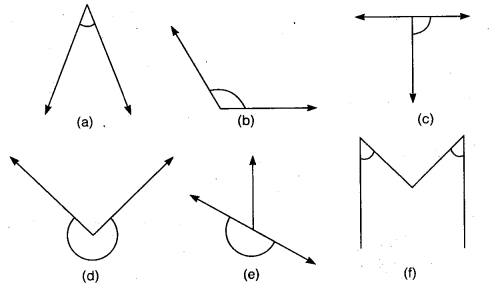# Class 6 Maths NCERT Solutions for Chapter 5 Understanding Elementary Shapes Ex – 5.3

## Understanding Elementary Shapes

Question 1.
Match the following:

(i) Straight angle                             (a) Less than one-fourth of a revolution
(ii) Right angle                                (b) More than half a revolution
(iii) Acute angle                              (c) Half of a revolution
(iv) Obtuse angle                          (d) One-fourth of a revolution
(v) Reflex angle                          (e) Between$\frac { 1 }{ 4 }$ and$\frac { 1 }{ 2 }$ of a revolution

Solution:
On matching, we find
(i) → (c)
(ii) → (d)
(iii) → (a)
(iv) → (e)
(v) → (b)

Question 2.
Classify each one of the following angles as right, straight, acute, obtuse or reflex:Solution:
Clearly, we find that
(a) → acute angle
(b) → obtuse angle
(c) → right angle
(d) → reflex angle
(e) → straight angle
(f) → acute angles.